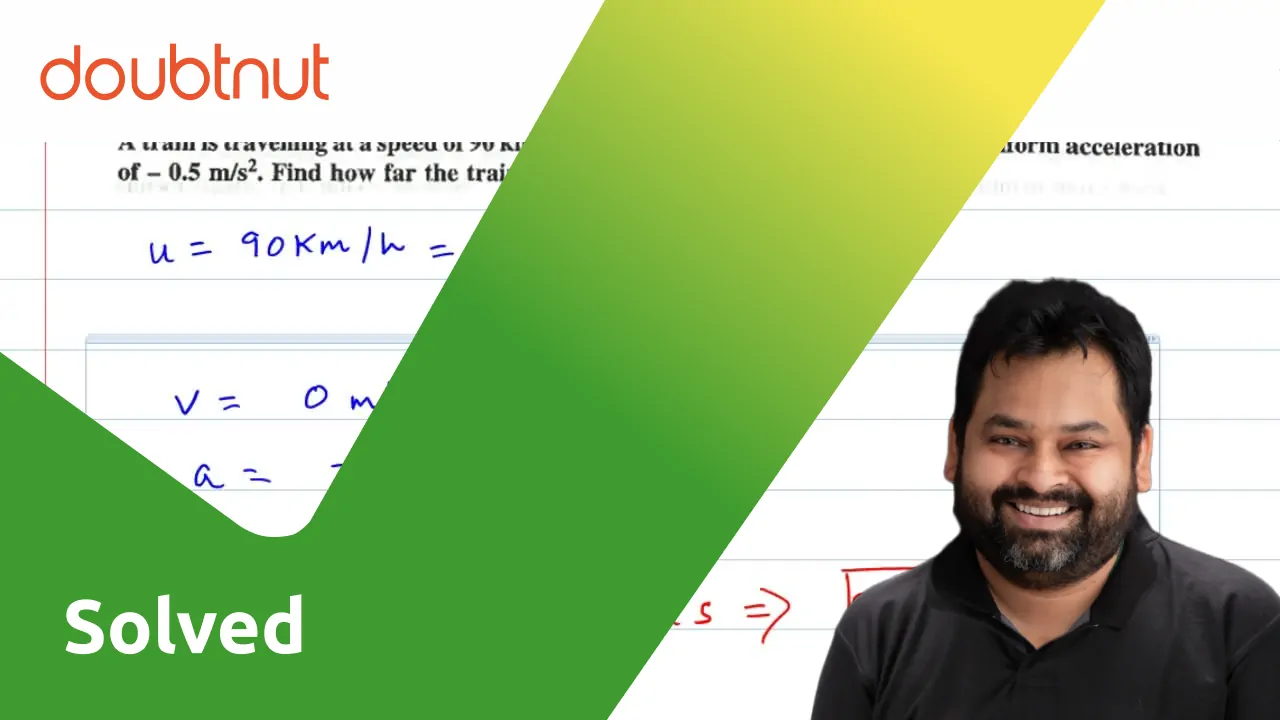Doubtnut App - Get 50 Lakh+ video solutions better than GoogleInstall Now

# A train is travelling at a speed of 90km/h. Brakes are applied so as to produce a uniform acceleration of −0.5m/s2. Find how far the train will go before it is brought to rest.

A

484 m

B

250 m

C

625 m

D

400 m

Text Solution
Verified by Experts

## Here, initial speed, u=90km/h−1=90×1000m60×60s=25m/s acceleration, a=−0.5m/s2, final velocity, v=0, distance travelled, s=? From v2−u2=2as, s=v2−u22a=0−(25)22×(−0.5)=625m